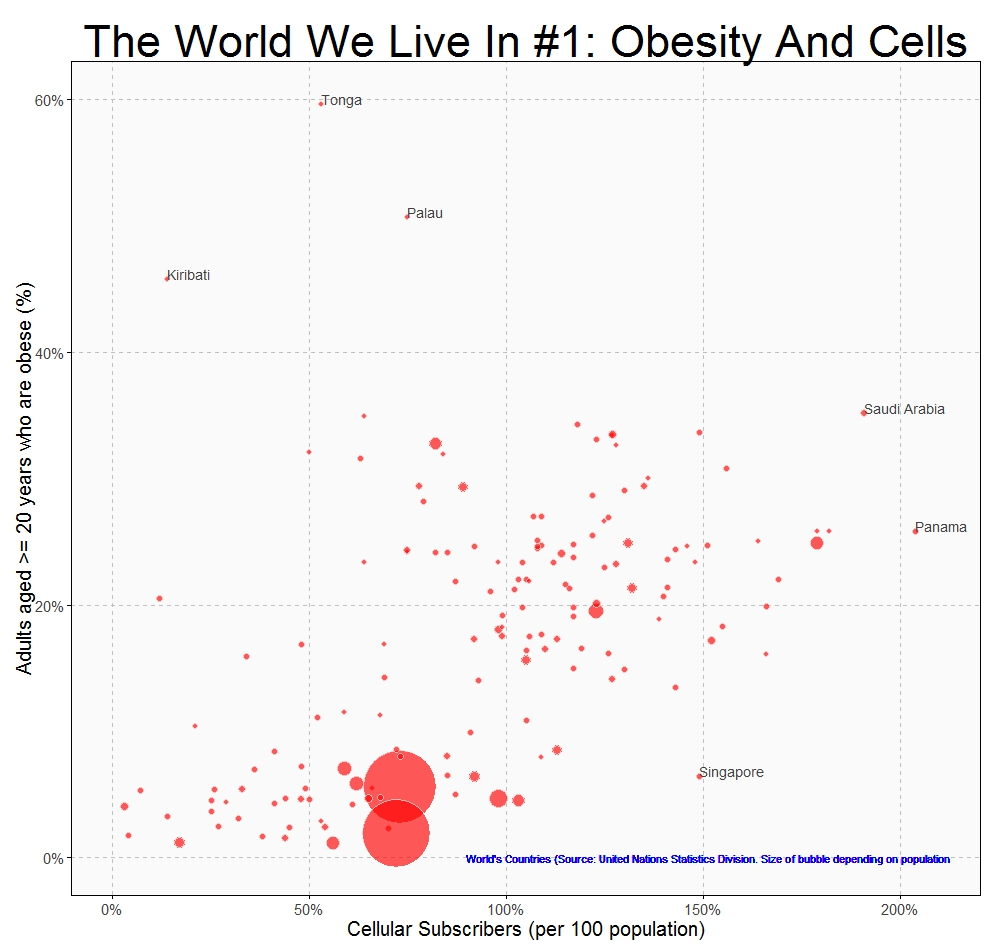# Watercolors

Do Sol de Ipanema
É mais que um poema
(Garota de Ipanema, João Gilberto)

Sometimes I think about the reasons why I spend so many time doing experiments and writing my discoveries in a blog. Even although the main reason to start this blog was some kind of vanity, today I have pretty clear why I still keep writing it: to keep my mind tuned. I really enjoy looking for ideas, learning new algorithms, figuring out the way to translate them into code and trying to discover new territories going a step further. I cannot imagine my life without coding. Many good times in the last years have been in front of my laptop listening music and drinking a beer. In these strange times, confined at house, coding has became in something more important. It keeps me ahead from the sad news and moves my mind to places where everything is quiet, friendly and perfect. Blogging is my therapy, my mindfulness.

This post is inspired in this post from Softology, an amazing blog I recommend you to read. In it, you can find a description of the stepping stone cellular automaton as well as a appealing collection of images generated using this technique. I modified the original algorithm described in the post to create images like these, which remind me a watercolor painting:

I begin with a 400 x 400 null matrix. After that, I choose a number of random pixels that will act as centers of circles. Around them I substitute the initial zeros by numbers drawned from a normal distribution which mean depends on the distance of pixels to the center. The next step is to apply the stepping stone algorithm. For each pixel, I substitute its value by a weighted average of itself and the value of some of its neighbors, choosen randomly. I always mix values of the pixels. The original algorithm, as described in the Softology’s blog, performs these mixings randomly. Another difference is that I mix values intead interchanging them, as the original algorithm does. Once I repeat this process a number of times, I pick a nice palette from COLOURLovers and turn values of pixels into colors with `ggplot`:

The code is here. Let me know if you do something interesting with it. Turning numbers into bright colors: I cannot imagine a better way to spend some hours in these shadowy times.

# The World We Live In #1: Obesity And Cells

Lesson learned, and the wheels keep turning (The Killers – The world we live in)

I discovered this site with a huge amount of data waiting to be analyzed. The first thing I’ve done is this simple graph, where you can see relationship between cellular subscribers and obese people. Bubbles are countries and its size depends on the population:Some quick conclusions:

• The more cellular subscribers, the more obese people
• Pacific islands such as Kiribati, Palau and Tonga are plenty of happy people
• Singapore people are thinner than they should be
• How do Saudi Arabian and Panamanian manage two cellulars?

This is the world we live in.

```cellular  =read.csv("UNdata_Export_20140930_cellular.csv",   nrows=193,   header=T, row.names=NULL)
require("sqldf")
require("plyr")
population=rename(population, replace = c("Country.or.Area" = "Country"))
population=sqldf("SELECT a.Country, a.Year, a.Value as Population
FROM population a INNER JOIN (SELECT Country, MAX(Year) AS Year FROM population GROUP BY 1) b
ON (a.Country=b.Country AND a.Year=b.Year)")
cellular=rename(cellular, replace = c("Country.or.Area" = "Country"))
cellular=rename(cellular, replace = c("Value" = "Cellular"))
obese=rename(obese, replace = c("Country.or.Area" = "Country"))
obese=rename(obese, replace = c("Year.s." = "Year"))
obese=sqldf("SELECT a.Country, a.Year, SUBSTR(TRIM(Value), 1, CHARINDEX(' [', TRIM(Value))) as Obeses
FROM obese a INNER JOIN (SELECT Country, MAX(Year) AS Year FROM obese WHERE GENDER='Both sexes' GROUP BY 1) b
ON (a.Country=b.Country AND a.Year=b.Year AND a.GENDER='Both sexes')")
obese\$Obeses=as.numeric(obese\$Obeses)
data=sqldf("SELECT a.Country, a.Cellular, c.Obeses, b.Population FROM cellular a inner join population b on a.Country = b.Country
inner join obese c on (a.Country = c.Country) WHERE a.Country NOT IN ('World', 'South Asia')")
require(ggplot2)
require(scales)
opts=theme(
panel.background = element_rect(fill="gray98"),
panel.border = element_rect(colour="black", fill=NA),
axis.line = element_line(size = 0.5, colour = "black"),
axis.ticks = element_line(colour="black"),
panel.grid.major = element_line(colour="gray75", linetype = 2),
panel.grid.minor = element_blank(),
axis.text.y = element_text(colour="gray25", size=15),
axis.text.x = element_text(colour="gray25", size=15),
text = element_text(size=20),
legend.key = element_blank(),
legend.position = "none",
legend.background = element_blank(),
plot.title = element_text(size = 45)
)
ggplot(data, aes(x=Cellular/100, y=Obeses/100, size=Population, label=Country), guide=FALSE)+
geom_point(colour="white", fill="red", shape=21, alpha=.65)+
scale_size_continuous(range=c(3,35))+
scale_x_continuous(limits=c(0,2.1), labels = percent)+
scale_y_continuous(limits=c(0,.6), labels = percent)+
labs(title="The World We Live In #1: Obesity And Cells",
x="Cellular Subscribers (per 100 population)",
y="Adults aged >= 20 years who are obese (%)")+
geom_text(data=subset(data, Cellular/100 > 1.9 | Obeses/100 > .4 | (Cellular/100 > 1.4 & Obeses/100 < .15)), size=5, colour="gray25", hjust=0, vjust=0)+
geom_text(aes(.9, .0), colour="blue", hjust=0, label="World's Countries (Source: United Nations Statistics Division. Size of bubble depending on population", size=4)+
opts
```